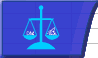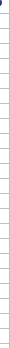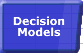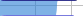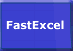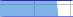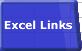# Totals and Conditional SumsImportance of Speed Faster Calculations Microsoft's Advice Bottlenecks/Size Lookups Dynamic Ranges Totalling Generating Workbooks Multi-Level Calculations Array Formulae SUMIF Example Worksheet Links## Totals and Conditional Sums

#### Totals as Final Results

If you need to produce totals and subtotals as part of the final results of your workbook try using Pivot Tables.

#### Totals as Intermediate Results

 Pivot Tables are a great way to produce summary reports, but try to avoid creating formulae that reference Pivot Table results. If you need to use totals and sub-totals as intermediate results in your calculation chain then it is not advisable to use Pivot tables unless you can ensure that: The Pivot table has been refreshed correctly during the calculation. The Pivot table has not been changed so that the information is still visible. If you still want to use Pivot Tables as intermediate results then use the GETPIVOTDATA function.

#### SUM and SUMIF:

Excel’s SUM and SUMIF functions are frequently used over a large number of cells.
Calculation time for these functions is proportionate to the number of cells covered.

#### Period-to-date and cumulative SUMs

There are two methods of doing period-to-date or cumulative SUMs:

Suppose the numbers you want to cumulatively SUM are in column A and you want Column B to have the cumulative sum.

You can create a formula in column B like =SUM(\$A\$1:\$A2) and drag it down as far as you need. The beginning cell of the SUM is anchored in A1, but because the finishing cell has a relative row reference it will automatically increase for each row.

Or you can create a formula like =\$A1 in cell B1 and like =\$B1+\$A2 in B2 and drag it down as far as you need. This calculates the cumulative cell by adding this rows number to the previous cumulative SUM.

For 1000 rows the first method makes Excel do about 500000 calculations, but the second method only makes Excel do about 2000 calculations (250 times more efficient!).

When you have multiple sorted indexes to a table (for instance City within Area) you can often save significant calculation time by dynamically calculating the address of a subset range of rows (or columns) to use in the SUM or SUMIF. See SUMIF Example or the FastExcel Sample problem for examples of this technique.

#### Wildcard SUMIF & COUNTIF

You can use the wildcard characters ? (any single character) and * (no character or any number of characters) as part of the SUMIF & COUNTIF criteria on alphabetic ranges.

### DSUM

Excel's DSUM function is very fast and uses a criteria range external to the function to specify multiple conditions for the sum. These criteria ranges allow great flexibility, but are difficult to use and maintain for large numbers of DSUM functions.

### Subtotals

#### Embedded Subtotals:

You can create embedded Subtotals using the Data -> Subtotals command on sorted data. I do not recommend using embedded subtotals because of the danger of inadvertent double counting.

#### The SUBTOTAL function.

 Use the SUBTOTAL function to SUM filtered Lists. The SUBTOTAL function is useful because, unlike SUM, it ignores: Hidden rows that result from filtering a list. In Excel 2003 only you can also make SUBTOTAL ignore all hidden rows, not just filtered out rows. Other SUBTOTAL functions.

#### Combined Subtotals and Totals.

If you are working with multidimensional data tables you often need to reference both subtotal and total intermediate results. Make sure you calculate the totals as a SUM of the subtotals rather than as a SUM of the whole table.© 2001-2020 Decision Models Legal Disclaimer Privacy Statement